# Oops...

This website is made for modern browsers. You are seeing this, because your browser is missing a feature or two. Please install the latest update or switch to a modern browser. See you soon.

# Generate lists

#lists

The methods presented below can be used to generate data objects in list format from given input parameters.

### Series of numbers (Series)

The component SeriesSeries (Series)
Sets  >  Sequence  >  Series
Create a series of numbers.
Inputs
Start (S)First number in the series
Step (N)Step size for each successive number
Count (C)Number of values in the series
Outputs
Series (S)Series of numbers
will generate a series of numbers. Input S determines the number to start with and input N represents the step size for each successive number. We can specify the number of values to be generated with input C.

### Series of characters (Char Sequence)

The component Char Sequence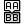Char Sequence (CharSeq)
Sets  >  Sequence  >  Char Sequence
Create a sequence of textual characters.
Inputs
Count (C)Number of elements in the sequence.
Char Pool (P)Pool of characters available to the sequence.
Outputs
Sequence (S)Sequence of character tags
is similar to Series, but will generate a series of characters. We can set the number of generated values at input C and set the sequence of available characters at input P, which is by default the alphabet. At Input F we can optionally provide additional string formatting: Axis {0} will become Axis A, Axis B, etc. Once all available characters are used up, they will be concatenated: X, Y, Z, AA, AB, AC, ….

### Subdivide number range (Range)

The component Range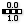Range (Range)
Sets  >  Sequence  >  Range
Create a range of numbers.
Inputs
Domain (D)Domain of numeric range
Steps (N)Number of steps
Outputs
Range (R)Range of numbers
will generate a list of numbers, not in a counting manner, but by subdividing a number range; the interval between two numbers will be equal. We set the domain of the range at input D and the number of steps at input N (8 steps will return 9 generated values).

### Generate random numbers (Random)

The component Random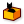Random (Random)
Sets  >  Sequence  >  Random
Generate a list of pseudo random numbers.
Inputs
Range (R)Domain of random numeric range
Number (N)Number of random values
Seed (S)Seed of random engine
Outputs
Random (R)Random numbers
will generate a list of random numbers. Input R sets the domain of possible numeric values and input N determines the number of generated items. We can influence the randomness by defining a seed.

By default, the generated numbers will have 6 decimal places. We can right-click the component and select the option Integer Number to only receive integers.

### Duplicates elements in a list (Duplicate Data)

The component Duplicate Data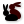Duplicate Data (Dup)
Sets  >  Sequence  >  Duplicate Data
Duplicate data a predefined number of times.
Inputs
Data (D)Data to duplicate
Number (N)Number of duplicates
Order (O)Retain list order
Outputs
Data (D)Duplicated data
will duplicate the data at input D a given number of times, which can be specified at input N. The default option is that the list is consecutively appended n-times.

At input O (Retain list order) we can influence the duplication procedure. If we switch from True to False, the list is no longer appended en bloc, but every element is duplicated n-times and before continuing with the next element.

### Repeat items in a list (Repeat Data)

The component Repeat Data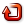Repeat Data (Repeat)
Sets  >  Sequence  >  Repeat Data
Repeat a pattern until it reaches a certain length.
Inputs
Data (D)Pattern to repeat
Length (L)Length of final pattern
Outputs
Data (D)Repeated data
will repeat the list items at input D. We can specify the number of items to be outputted at input L. Unlike Duplicate Data, which duplicates the input list as a whole, we can now define the length of the emitted list. This does not have to be a multiply of the input list.

The items at input D will be repeated, if the requested length exceeds the number of provided items. If we provide the alphabet as input data, then after Z will follow A. This way, we achieve a different behavior compared to how Char SequenceChar Sequence (CharSeq)
Sets  >  Sequence  >  Char Sequence
Create a sequence of textual characters.
Inputs
Count (C)Number of elements in the sequence.
Char Pool (P)Pool of characters available to the sequence.
Outputs
Sequence (S)Sequence of character tags
operates.

### Multiply specific items in a list (Stack Data)

The component Stack Data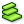Stack Data (Stack)
Sets  >  Sequence  >  Stack Data
Duplicate individual items in a list of data
Inputs
Data (D)Data to stack
Stack (S)Stacking pattern
Outputs
Data (D)Stacked data
is similar to Duplicate Data on which input O (Retain list order) set to False: We can provide data to duplicate at input D and the number of duplicates at input S. Unlike before, input S can now be a list and states the number of duplications for each index. This allows us to fine tune how many duplicates we need for each item.

### Generate a sequence of numbers (Sequence)

The component Sequence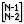Sequence (Seq)
Sets  >  Sequence  >  Sequence
Generate a sequence of numbers
Inputs
Notation (N)Sequence notation
Length (L)Final length of sequence
Initial (I)Initial values in sequence
Outputs
Sequence (S)Sequence
will generate a sequence according to a notation given at input N. Input L determines the number of generated values and input I sets the initial values for the sequence.

To illustrate the example shown in the image above, we use the notation [N-1] + [N-2] together with two initial values (here 0 and 1). The first two values of our generated list are determined by the initial values and thus are 0 and 1. The third value is calculated by adding the last value N-1 and the value before last [N-2]. Continuing, this gives:

0:   0     initial value
1:   1     initial value
2:   1     (1 + 0)
3:   2     (1 + 1)
4:   3     (2 + 1)
5:   5     (3 + 2)
6:   8     (5 + 3)
7:   13    (8 + 5)


This sequence is better know as Fibbonacci sequence and Grasshopper offers a dedicated component to calculate this special sequence: Fibonacci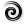Fibonacci (Fib)
Sets  >  Sequence  >  Fibonacci
Creates a Fibonacci sequence.
Inputs
Seed A (A)First seed number of the sequence
Seed B (B)Second seed number of the sequence
Number (N)Number of values in the sequence
Outputs
Series (S)First N numbers in this Fibonacci sequence
.

Besides simple mathematical operators, we can also provide more complex expressions

as input for SequenceSequence (Seq)
Sets  >  Sequence  >  Sequence
Generate a sequence of numbers
Inputs
Notation (N)Sequence notation
Length (L)Final length of sequence
Initial (I)Initial values in sequence
Outputs
Sequence (S)Sequence
. For example, [N-1] + If([N-1]%2=0,1,9) will add the value of 1 to every even number and 9 to every uneven.

This page is open source. Edit it on GitHub or see how you can contribute.